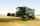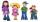Unknown number 10

Number first increased by 30%, then by 1/5. What percentage we've increased the original number?

Result

p =  50 %

Solution:

1 + 30/100 + 1/5 = 1+p/100

p = 50

p = 50

Calculated by our simple equation calculator.

Leave us a comment of this math problem and its solution (i.e. if it is still somewhat unclear...):Be the first to comment!To solve this verbal math problem are needed these knowledge from mathematics:

Our percentage calculator will help you quickly calculate various typical tasks with percentages. Do you have a linear equation or system of equations and looking for its solution? Or do you have quadratic equation?

Next similar math problems:

1. Two numbersWe have two numbers. Their sum is 140. One-fifth of the first number is equal to half the second number. Determine those unknown numbers.
2. Reducing numberReducing the an unknown number by 28.5% we get number 243.1. Determine unknown number.
3. Four numbersThe first number is 50% second, the second number is 40% third, the third number is 20% of the fourth. The sum is 396. What are the numbers?
4. Jane classWhen asked how many students are in class, Jane said, if we increase the number of students in our class by hundred % and then add half the number of students, we get 100. How many students are in Jane's class?
5. Perctentages35% of what number is 35?
6. Walnutsx walnuts were in the mission. Dano took 1/4 of nuts Michael took 1/8 from the rest and John took 34 nuts. It stayed here 29 nuts. Determine the original number of nuts.
7. PercentsHow many percents is 900 greater than the number 750?
8. Football match 4In a football match with the Italy lost 3 goals with Germans. Totally fell 5 goals in the match. Determine the number of goals of Italy and Germany.
9. The largerThe larger of two numbers is nine more than four times the smaller number. The sum of the two numbers is fifty-nine. Find the two numbers.
10. Frameworks is badCalculate how many percent will increase the length of an HTML document, if any ASCII character unnecessarily encoded as hexadecimal HTML entity composed of six characters (ampersand, grid #, x, two hex digits and the semicolon). Ie. space as: &#x20;
11. The farmerThe farmer harvested 840 tons of grain in 2006, which was 44% less than in 2005, and one-fifth more than in 2004. How many tons of grain harvested in years 2005 and 2004?
12. School yearAt the end of the school year has awarded 20% of the 250 children who attend school. Awat got 18% boys and 23% of girls. Determine how many boys and how many girls attend school.
13. MushroomsEva and Jane collected 114 mushrooms together. Eve found twice as much as Jane. How many mushrooms found each of them?
14. Three friendsThe three friends spent 600 KC in a teahouse. Thomas paid twice as much as Paul. Paul a half less than Zdeněk. How many each paid?
15. EquationsSolve following system of equations: 6(x+7)+4(y-5)=12 2(x+y)-3(-2x+4y)=-44
16. Rabbits 3Viju has 40 chickens and rabbits. If in all there are 90 legs. How many rabbits are there with Viju?
17. TreesAlong the road were planted 250 trees of two types. Cherry for 60 CZK apiece and apple 50 CZK apiece. The entire plantation cost 12,800 CZK. How many was cherries and apples?